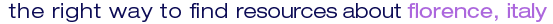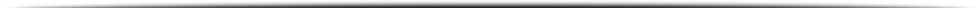# florence italy news by the florence newspaperNEWS FROM FLORENCE, ITALY ∼ PROVIDED BY THE FLORENCE NEWSPAPER

<% function dataora(codice) dim vet, annu, str dataora="" vet = split(codice,".") select case vet(0) case "97" annu = "97" case "98" annu = "98" case "99" annu = "99" case "a0" annu = "00" case "a1" annu = "01" case "a2" annu = "02" case "a3" annu = "03" case "a4" annu = "04" case "a5" annu = "05" case "a6" annu = "06" case "a7" annu = "07" case "a8" annu = "08" case "a9" annu = "09" case else annu = "" end select str = vet(2) & "/" & vet(1) & "/" & annu & "  " & vet(3) & ":" & vet(4) dataora=str end function Function HTMLDecode(sText) Dim I 'Originale 'sText = Replace(sText, """, Chr(34)) 'sText = Replace(sText, "<" , Chr(60)) 'sText = Replace(sText, ">" , Chr(62)) 'sText = Replace(sText, "&" , Chr(38)) 'sText = Replace(sText, " ", Chr(32)) 'modificato da cardelli sText = Replace(sText, """, Chr(32)) sText = Replace(sText, "<" , Chr(32)) sText = Replace(sText, ">" , Chr(32)) sText = Replace(sText, "&" , Chr(32)) sText = Replace(sText, " ", Chr(32)) For I = 1 to 255 sText = Replace(sText, "&#" & I & ";", Chr(I)) Next HTMLDecode = sText End Function 'create object Dim rss Set rss= New RSSContentFeed 'get content rss.ContentURL = "http://www.florencenewspaper.it/xmlexport/rssfull.asp?Florence=1&Events=1&Life=1&howmany=50" rss.GetRSS() Dim i For Each i in rss.Results %>